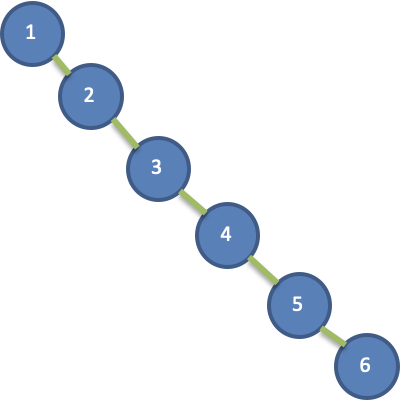# 数据结构：二叉树

## 树的诞生

1、数组存储方式的分析

• 数组未排序

• 优点：在数组尾添加元素，速度快

• 缺点：查找速度慢

• 数组排序

• 优点：可以使用二分查找，查找速度快
• 缺点：为了保证数组有序，在添加新数据时，找到插入位置后，后面的数据需整体移动，速度慢。

2、链式存储方式的分析

• 优点：在一定程度上对数组存储方式有优化，增加和删除元素速度快
• 缺点：不管链表是否有序，查找速度都慢(检索某个值，需要从头节点开始遍历)

3、树存储方式的分析

## 树中的术语

• 结点：指树中的一个元素，一般用 n 表示

• 结点的度：指结点拥有的子结点的个数，二叉树的度不大于2

• 树的度：指树中各结点的度的最大值

• 树的阶：指树中的一个结点最多能有多少个子节点，一般用字母 M 表示阶数

• 分支结点：度大于0的结点，除了叶子结点之外的结点

• 叶结点：度为 0 的结点，也称为终端结点

• 高度：从下到上

• 指从叶子节点到该节点的最长简单路径边的条数或者节点数

• 叶子节点的高度为1，根节点高度最高

• 深度：从上到下

• 指从根节点到该节点的最长简单路径边的条数或者节点数

• 根节点的高度就是二叉树的最大深度

• 层：规定根节点在1层，其他任意节点是其父节点的层数+1

• 森林：彼此不相交的树的集合

## 二叉树

### 简介

• 二叉树：每个结点最多只能有两个子结点(左结点和右结点)
• 满二叉树：二叉树的所有叶子结点都在最后一层，且结点总数为 2n -1 , n 为层数
• 完全二叉树：二叉树的所有叶子结点都在最后一层或者倒数第二层，而且最后一层的叶子结点在左边连续，倒数第二层的叶子结点在右边连续
• 左斜树：所有节点都只有左子树的二叉树
• 右斜树：所有节点都只有右子树的二叉树

### 前序遍历

#### 递归

``````public void preOrderRecur(Node root) {
if (root == null) {
return;
}
System.out.print(root.data + " -> ");
preOrderRecur(root.left);
preOrderRecur(root.right);
}
``````

#### 非递归

``````public void preOrder() {
if (root == null)
return;
Node current;
while (!s.isEmpty()) {
current = s.removeFirst();
System.out.print(current.data + " -> ");
if (current.right != null)
if (current.left != null)
}

}
``````

### 中序遍历

#### 递归

``````public void inOrderRecur(Node root) {
if (root == null) {
return;
}
inOrderRecur(root.left);
System.out.print(root.data + " -> ");
inOrderRecur(root.right);
}
``````

#### 非递归

``````public void inOrder() {
Node current = root;
while (current != null || !s.isEmpty()) {
while (current != null) {
current = current.left;
}
if (!s.isEmpty()) {
current = s.removeFirst();
System.out.print(current.data + " -> ");
current = current.right;
}
}
}
``````

### 后序遍历

#### 递归

``````public void postOrderRecur(Node root) {
if (root == null) {
return;
}
postOrderRecur(root.left);
postOrderRecur(root.right);
System.out.print(root.data + " -> ");
}
``````

#### 非递归

``````public void postOrder() {
Node current = root;
while (current != null || !s1.isEmpty()) {
while (current != null) {
current = current.right;
}
if (!s1.isEmpty()) {
current = s1.removeFirst();
current = current.left;
}
}
while (!s2.isEmpty()) {
System.out.print(s2.removeFirst().data + " -> ");
}
}
``````

### 层次遍历

``````void bfs(TreeNode root) {
Queue<TreeNode> queue = new ArrayDeque<>();
while (!queue.isEmpty()) {
TreeNode node = queue.poll();
if (node.left != null) {
}
if (node.right != null) {
}
}
}
``````

### 查找

``````public boolean contains(int e) {
//用current记录根节点
Node current = root;
while (current != null) {
//相等，说明找到了，返回true
if (e == current.data) {
return true;
//要查找的数比根节点大，指向根节点的右儿子
} else if (e > current.data) {
current = current.right;
//要查找的数比根节点下，指向根节点的左儿子
} else {
current = current.left;
}
}
//找不到，返回false
return false;
}
``````

### 删除

``````
``````

。。。

。。。

## 二叉搜索树-BST

。。。

。。。

### 复杂度

• 时间复杂度：O(log2n)～O(n)

• 如果二叉搜索树是平衡的，则 n 个节点的二叉排序树的高度为 log2n+1 ，其查找效率为O(log2n)，近似于折半查找。

• 如果二叉搜索树完全不平衡，则其深度可达到 n，查找效率为 O(n)，退化为顺序查找。

• 空间复杂度：O(n)

## 平衡二叉树-AVL

### 引出• 插入速度没有影响
• 查询速度明显降低(需要依次比较)，搜索效率降低为 O(n)

### 简介

• 必须是二叉搜索树
• 每个结点的左子树和右子树的高度差的绝对值不超过1

### 左旋转### 右旋转。。。

## 红黑树

• 结点不是红色就是黑色，根结点必是黑色

• 叶子结点是 null 结点（空结点）且为黑色

• 每个红色结点的两个子结点都是黑色。（同一路径，不存在连续的红色节点）

• 每个节点到叶子节点的所有路径，都包含相同数目的黑色节点（相同的黑色高度）

。。。

## 参考

 支付宝支付 微信支付上一篇数据结构：B-Tree家族
B-tree 论文名称：《Organization and maintenance of large ordered indices Share on》 作者：R. Bayer，E. McCreight 单位：Mathematical
2022-04-11JavaSE-汇总

2022-04-10
目录